# Landscape change analysis with MOLUSCE - methods and algorithms

Перейти к: навигация, поиск
Эта страница является черновиком статьи.

The plugin can be installed from QGIS Python Plugins Repository.

Source code of the plugin is hosted on github.

A simple user guide can be installed with the plugin or viewed on github.

 Создано в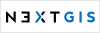Веб ГИС для вашей организации по доступной цене

The plugin is developed by NextGIS in cooperation with Asia Air Survey, Japan.

## Terminology used in this document

• MOLUSCE is a QGIS plugin for Land Use Change Evaluation. MOLUSCE abbreviation stands for Methods Of Land Use Chande Evaluation. Briefly, the plugin implements the following process:
1. Takes raster of land use categories for time period A (past), raster of land use categories for the time period B (present) and rasters of explanatory variables or factors.
2. Trains a model that predicts land use changes from past to present.
3. Predicts future land use changes using derived model, current state of land use and current factors.
• Model is an algorithm that is used for prediction of land use changes (transitions).
• State raster is one-band raster where each pixel is assigned with landuse category.
• Input state raster is one-band raster describing the past.
• Output state raster is one-band raster describing present. It is the desired result of prediction for model (a user trains the model to predict (input raster, factors) -> output raster).
• Factor raster is a raster of explanatory variables. The rasters can be one-band or multi-band.
• Transition class or simple 'transition' is a information about land use change. Every change (for example forest -> non-forest) can be viewed as a transition of land use categories (encoded as pixels) from Input state raster to Output state raster.
• Change map is a integer one-band raster that stores information about transitions. Category values of change map are mapped one-to-one to transition classes.

## General structure of the plugin

MOLUSCE consists of several parts. The most important are:

• GUI modules - implement user interface
• Utility modules
• Data Provider - provides procedures for reading/writing raster data and similar utility functions
• Cross Tabulation - provides functions for creating contingency tables
• Sampler - provides sampling procedure
• Algorithmic modules:
• Area Analysis - provides procedures to calculate amount change between states, create change maps
• Modeling - provides submodules for modeling relation between input-output data
• Simulation - provides procedure of land change simulation
• Validation - provides statistic functions and procedures for validation of simulation result

This manual gives general overview of the modules. The description is valid for structure of plugin version 3.x.x

## Utility modules

Utility modules are called from other modules of the plugin. The most important utility modules such as DataProvider and Sampler are discussed in this section.

### Data Provider

This module provides data structure for internal storing of raster data. It uses numpy masked arrays as data store. Data Provider provides methods for:

• Creation and storing rasters: reading data from file and manage no-data values, creating new rasters, saving data.
• Access to the data and data manipulation: Read particular raster band, replace a band by new array of pixels, get statistics of raster's bands, normalize the data for particular algorithm, reads geometry and geography related information from the raster variable, etc.
• Comparing geodata objects: check raster sizes, projections and geo transform objects between rasters.

### Sampler

Sampler is the module that performs sampling procedure. A sample is a set of input data and corresponding output data that has to be predicted via a model.

#### Initialization of sampling procedure

Sampling procedure is used by Logistic regression and Multilayer perceptron predictors. This procedure collects samples that are used during fitting of the model. But Sampler collects transformed data, because usually the transformation allows achieve better fitting. MOLUSCE uses two transformations:

• Dummy coding for categorical variables.
• Normalization for continuous and ordered variables.
##### Dummy coding

As the initial state raster and change map are categorical, each of them must be transformed to set of independent variables. To do that the plugin uses dummy coding - a categorical variable of N levels is divided into N-1 dummy variables. For example, for 4 classes land use map (Agriculture, Forest, Urban, Water), we have to use 3 dummy variables:

 Dummy 1 Dummy 2 Dummy 3 Agriculture 0 0 1 Forest 0 1 0 Urban 1 0 0 Water 0 0 0

One row (in this case Water) consists of zeros. It is important because otherwise the dummy variables became linear-dependent. The condition of linear independence of data is crucial for Logistic regression and strongly recommended for Multilayer Percepton.

##### Normalization

The next stage of initialization procedure is normalization of factor data. Usually normalization allows to achieve more efficient training and more accurate prediction result. Plugin uses linear normalization: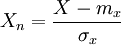$X_n = \frac{X-m_x}{\sigma_x}$

where X is a variable, Xn is normalized variable, mx is mean of X and σx is standard deviation of X.

#### Description of the sampling process

Sampler works with single pixels and a neighborhood of pixels. This means that a user can take into account a set of pixels around current pixel. The implementation of the algorithm uses Moore neighborhood. For example, if the user sets up 1-size neighborhood then sampling procedure reads the current pixel and all pixels located in immediate vicinity (3x3 window size). If the user set up 2-size neighborhood then sampling procedure reads the current pixel and all pixels located in 5x5 window size and so on. Every neighborhood is stored as vector (9 elements, 25 elements and so on). Sampling is performed for every input raster (initial state raster and factors).

Thus a sample contains:

• coordinates of the pixel,
• input data (consists of 2 parts):
• state pixel vector is the neighborhood that is read from 1-band raster, this raster contains initial states (categories). The raster is divided into set of dummy variables before sampling (see below).
• factor pixel vectors are the neighborhoods extracted from factor rasters (they can be multiband).
• output data is read from 1-band raster, this raster contains final states.

There are several sampling modes:

• "All". This mode extracts information for all pixels of the input and output rasters. Among other methods this mode of sampling needs the biggest amount of RAM and CPU time.
• "Random". This mode extracts information for randomly selected samples: if raster has N pixels, then the every pixel has 1/N probability of being selected.
• "Stratified". This mode randomly undersamples major categories (large areas) and/or oversamples minor categories (small areas). If C is desired count of samples and K is number of output categories, then the sampling procedure select K random groups of pixels of equal size C/K. Every group will contain pixels of one certain category.

### Cross Tabulation

Cross Tabulation is a module that is used in several places of the workflow. The main purpose of the module is to create contingency tables. For example it is used during creating of transition matrix. In this case the method calculates transition statistic -- how many pixels has been changed. The result is the table:

 Category 1 Category 2 ... Category N Category 1 Count of unchanged pixels of Category 1 Count of transition Category 1 - > Category 2 ... Count of transition Category 1 - > Category N Category 2 Count of transition Category 2 - > Category 1 Count of unchanged pixels of Category 2 ... Count of transition Category 2 - > Category N ... ... ... ... ... Category N Count of transition Category N - > Category 1 Count of transition Category N - > Category 2 ... Count of unchanged pixels of Category N

## Algorithmic modules

### Area Analysis

The main purpose of area analysis module is change map calculation. The module uses the next scheme of transition encoding:

 Category 1 Category 2 ... Category N Category 1 0 1 ... N -1 Category 2 N N + 1 ... 2N -1 ... ... ... ... ... Category N (N-1)*N (N-1)*N + 1 ... N*N -1

For example, if in the state rasters category "Forest" coded as "3", category "Non-forest" coded as "2", then transition "forest" -> "non-forest" in the change map will be coded as "7".

For example, for 4 classes (N=4), there are 4*4=16 possible transitions:

 Agriculture Forest Urban Water Agriculture 0 1 2 3 Forest 4 5 6 7 Urban 8 9 10 11 Water 12 13 14 15

Output of Area Analysis stage is a change map - raster where each pixel is assigned an integer value indicating its transition.

### Modeling

A user has a number of factors, init state raster and final state raster. The goal of modeling stage is to create a model that can predict land use changes between those states. Before discussing prediction methods we need to explain common preparation stage.

The first subsection of this section discuses types of input and output data and necessary manipulations with the data, the next subsections describe concrete realizations of predicting models.

#### Data types and initialization procedures

The next assumptions are currently used by the plugin:

• Initial and final state rasters are categorical one-band rasters, therefore a change map is categorical one-band raster too.
• For ANN, MCE and LR model factor rasters assumed ordinal or continuous (one-band or multiband), but not categorical.

#### Multilayer perceptron

The plugin uses classic realization of Multilayer perceptron. The input data is a collection of pixels of initial state raster and factor rasters. The target output data is the change map.

So, the perceptron model performs the next actions:

1. Initial preprocessing of the data (dummy coding and normalization).
2. Sampling.
3. Training.

The initial stages are described (in general) in the previous section, this subsection discussed the structure of the neural net, the training procedure and particular features of initialization.

MOLUSCE uses multilayer percepron with numpy.tanh sigmoid function. Therefore target variables (the change map categories) should be scaled to (-1, 1) interval during dummy coding instead (0, 1). A user can set arbitrary number of hidden layers (one or more) and arbitrary number (one or more) of neurons in the layers.

The created net has

• (C − 1)(2N + 1)2 + B(2N + 1)2 input neurons;
• M output neurons (it depends on samplig mode);

Where C is the count of land use categories, N is the neighborhood size specified by the user, B is the summary band count of factor rasters (for example if two factors are one-band and three-band, then B=4). M usually is a count of uniques categories in the change map (C2). But the count of output neurons can be less then M, it depends on the sampling mode. If mode "Random" is used, some small classes could be omitted during sampling procedure.

The module uses classic backpropagation algorithm with momentum for the learning procedure. Weights correction are performed as

w(n + 1) = r * dw(n) + m * dw(n − 1)

where w is a vector of neuron weights, dw is a vector of weights changes, n is an iteration number, r is learning rate, m is momentum.

Training set is divided in two parts: learning set (80% of samples by default) and validation set (20% of samples).

The module uses on-line learning with stochastic: a random sample is selected from the learning set, the weights of the net are updated during forward/backward propagation.

A error of fitting (for a sample) is the average square error of partial outputs of the net:$E = \frac{t_i-o_i}{d}$

where E is a sample error, ti is the target value of a output neuron for given sample, oi is the real output value of the neuron, d is the count of output neurons.

The module returns the next information about learning:

• Graph of the average square errors. The errors (mean of all E on learning set and validation set) are calculated on learning and validation sets after every epoch and plotted on the graph.
• Min validation overall error. This is average square error E that is achieved on all validation set (mean of all E on validation set). This is the best currently achieved result. The weights of the net are stored and after training the best weights will be returned as the best net.
• Delta overall accuracy. It is the difference between min validation error and current validation error.
• Current validation kappa. It is the kappa statistic achieved on the validation set.

#### Logistic regression

Logistic regression model behavior is very similar to the ANN. The input data is a collection of pixels of initial state raster and factor rasters. The target output data is the change map.

So, the logistic regression model also performs the next actions:

1. Initial preprocessing of the data (dummy coding and normalization).
2. Sampling.
3. Training.

The created model has M((C − 1)(2N + 1)2 + B(2N + 1)2 + 1) coefficients, but only (M − 1)((C − 1)(2N + 1)2 + B(2N + 1)2) are independent. As before (see perceptron discussion), C is the count of land use categories, N is neighborhood size specified by the user, B is summary band count of factor rasters (for example if two factors are user one-band and three-band, then B=4). M usually is count of uniques categories in the change map (C2). M depends on the sampling mode also.

For example, discuss coefficients of one transition class (transition from land use category 2 to land use category 3, for example). If we have four land use categories 1,2,3,4 and we select one one-band factor and we use 0-neighborhood, we have 5 coefficients per transition class:

• b0 is an intercept;
• b1,b2,b3 are the coefficients of dummy variables of current landuse state (categories of the raster are coded as: 1 = (0, 0, 1); 2 = (0, 1, 0); 3 = (1, 0, 0); 4 = (0, 0, 0), see Sampler discussion);
• b4 is the coefficient of factor pixel (0-neighborhood).

If we select two one-band factors, we have 6 coefficients per transition class:

• b0 is an intercept;
• b1,b2,b3 are the coefficients of dummy variables of current landuse state;
• b4 is the coefficient of the first factor (0-neighborhood).
• b5 is the coefficient of the second factor (0-neighborhood).

if we use 1-neighborhood and two one-band factors, we have 37 coefficients per transition class:

• b0 is an intercept;
• coefficients from b1 thru b27 are the coefficients of dummy variables of current landuse state: (b1, ..., b3 are dummy variables for the upper-left corner of 3x3 neighborhood window);
• coefficients from b28 thru b36 are the coefficients of the first raster pixels (1-neighborhood uses 9 pixels or 3x3 window);
• coefficients from b37 thru b45 are the coefficients of the second raster pixels.

#### Weight of evidence

Weight of evidence method was developed to process binary maps, but the method was later modified in order to enable it to handle multiple categories of variables. If user uses rasters with continious values, he has to transform them into categorical variables. MOLUSCE implementation uses the last one modification. To handle continiou factors user must split raster values into several categories (range binning). The GUI has a form that allows to perform transformation into categorical variables. A compromise is needed here: if the user set up too many binning values, then a small amount of pixels might fall into a category and accuracy of statistical calculations will be poor; on contrary if the user set up few bins, which will lead to few of weights are formed, that leads to poor accuracy again.

In the binning form GUI doesn't display all of the used rasters: if a raster is already categorical, it doesn't need transformation and it doesn't appear in the form. Currently a simple procedure is used to decide if rasters as categorical: if a raster has more then MAX_CAT (=100) unique values, then the raster is supposed to be continious, otherwise the raster is treated as categorical.

The WoE fitting procedure starts after the transformation continious -> categorical.The change map is divided into series of binary maps (one map per a transition class), then the set of weights are estimated for every binary map.

#### Multi criteria evaluation

The goal of the method is to predict a transition class encoded in the change map. Factor rasters are input data, places where a transition occurs are target values. MCE takes pairwaise comparison matrix of factors and calculates weights of every factor. This means, that a user must specify the comparison matrix for every transition class. But count of transition classes increases as O(n2), where n is count of landuse categories (for example if n=4, we have 16 transition classes). To specify all comparison matrices for all transitions is too long and boring, that's why the MCE model predicts one transition class only.

The method needs normalization of factor rasters: this implementation uses a linear combination of factors, therefore they should be transformed to [0,1]. The module uses the next transformation: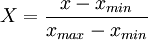$X = \frac{x - x_{min}}{x_{max}- x_{min}}$

where X is normalized raster value, x is initial raster value, xmin is the min value of the raster, xmax is the max value of the raster.

### Simulation

Simulator module performs land use change evaluation procedure. It takes as input the next data:

• Initial state raster;
• Factor rasters;
• Model.

Initial state raster contains information about current land use categories, factor rasters contain information about explanatory variables. Model is a predictor that calculates transition potentials in the condition of the factors and current land use. So module doesn't uses implicit transition rules, it uses transition potentials generated by the models. The neighborhood effect is achieved if a model uses neighborhood during training, for example logistic regression has a coefficient for every neighbor and the coefficient affects the transition potential. If model doesn't uses neighborhood, Simulator takes into account only general patterns.

The module works as follow scheme:

1. Simulator takes transition probabilities from the transition matrix and calculates count of pixels that has to be changed (for every transition class);
2. Simulator calls a model, pass to it initial state raster and factor rasters;
3. The model scans pixels of the rasters (and its neighbors if the model uses neigborhood) and calculates transition potentials of every transition class;
4. Simulator constructs a 'certancy' raster: the pixel of the raster are the difference between two most large potentials of correspondent pixels of transition potential rasters. As result the raster contains the model confidence: the bigger is difference, the bigger is confidence;
5. Simulator constructs a raster of the most probable transitions: the pixels of the raster are the transition class with the biggest potential of transition. This raster is an auxiliary raster used during the next stage;
6. For every transition class Simulator searches in the raster of the most probable transitions a needed count of pixels with the greatest confidence and changes the category of the pixels. If confidence of two or more pixels are close, then random choise of the pixel is used.

The module gives the next outputs:

• Rasters of transition potential (scaled to percents) that are output from the models;
• Raster of the difference between the two most large potentials or 'certancy';
• Result of simulation.

The described scheme is used if a user specified only one iteration of simulation. If several iterations have to be preformed, in general the scheme is the same, but initial state rasters are changed on the next iterations: the first simulated map is the initial state map for the second iteration, the result of the second iteration is the initial states of the third iteration and so on.

### Validation

Validation module allows to check accuracy of the simulation. MOLUSCE has three types of validation:

• Kappa statistics;
• Error budget validation;
• Error map validation.

#### Kappa

The module calculates three types of kappa statistic:

1. Kappa: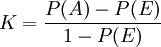$K = \frac{P(A)-P(E)}{1-P(E)}$
2. Kappa location: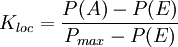$K_{loc} = \frac{P(A) - P(E)}{P_{max}-P(E)}$
3. Kappa histogram: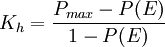$K_{h} = \frac{P_{max} - P(E)}{1-P(E)}$

where$P(A) = \sum_{i=1}^c p_{ii}$,$P(E) = \sum_{i=1}^c p_{iT}p_{Ti}$,$P_{max} = \sum_{i=1}^c min(p_{iT}, p_{Ti})$ and pij is the i,j-th cell of contingency table, piT is the sum of all cells in i-th row, pTj is the sum of all cells in j-th column, c is count of raster categories.

#### Error budget

MOLUSCE uses Error Budget technique for validation. The article propose 15 types of statistic, but MOLUSCE calculates and plots 5 of the most important of them:

• No location information, no quantity information;
• No location information, medium quantity information;
• Medium location information, medium quantity information;
• Perfect location information, medium quantity information;
• Perfect location information, perfect quantity information.

The used scale denominator is two, that means that every iteration the module increases the pixels area of reference and comparison rasters in four times.

#### Error map

MOLUSCE produces map of errors. This map contains information about false predictions in simulated raster. The error map can be constructed by compassion of simulated map and reference map. The errors of simulation are stored in the error map.

Error map creating procedure has two modifications: two map comparison case and three map comparison case.

##### Two maps comparison case

This modification compares simulated map and reference map.

Traditional approach to two class (C1 and C2) problem uses tree types of prediction statuses:

 Reference Map C1 Reference Map C1 Simulated C1 Hit Misses Simulated C2 False alarms Hit

In common case (N classes) count of the possible types of errors is rather large, for example if three land use classes are analyzed, then there are 9 possible transition classes and there are three hits in the table:

 Reference Map C1 Reference Map C1 Reference Map C3 Simulated C1 Hit Error (type 12) Error (type 13) Simulated C2 Error (type 21) Hit Error (type 23) Simulated C3 Error (type 31) Error (type 32) Hit

So in case of N class problem the classification "hits, misses, false alarm" is not so good because the terms "Misses" and "False Alarms" became ambiguous.

MOLUSCE uses common N class approach, when error map contains every transition type error marked by a particular color, "Hits" are transparent. The errors are coded as transitions in change maps (see Area Analysis section).

An example of error map for land use classes "Forest", "Agriculture", "Urban" and "Pasture" is presented on the picture below.

This picture contains location of error pixels. If a transition for a certain location was simulated correctly then the pixel is transparent (white color on the picture). If the transition was simulated incorrect, then the pixel is colored. For example a big cloud of light-green pixels is located on the right side of the error map, the color says that the pixels mark locations of false "Urban to Pasture" transition. This means that in the reference map the pixel has "Urban" meaning but in the simulated map the pixel is "Pasture". A similar interpretation can be done for other colors.

#### Three map comparison case

This modification uses three rasters for checking: simulated map, reference map and initial map.

The same idea of comparison is used for checking persistent classes and simulation errors. Persistent classes are pixels that are constant in initial raster, reference raster and simulated raster. Such pixels are marked by a certain color, "Hits" are transparent and transition errors are marked as in the two-map comparison problem.

## Special topics

### Memory Usage

This section contains some examples of the plugin memory usage. The table lists stage of analysis and approximate memory usage (reported by system resource manager). Column Memory shows information about peak memory usage achieved during the stage; later stages can accumulate memory usage from previous stages (for example simulation stage needs data of model stage), but after completing a stage some of unused memory can be released. This process can be viewed in the tables.

The table contains data about tree landuse class task and three one-band factors, the raster sizes are approximately 2000x2500 pixels. In the table ANN has one hidden layer of 10 neurons. The models are trained on 2500 samples, neighborhood is 1.

 Stage Peak memory usage (Mb) Reading rasters 45 Correlation checking 375 Updating transition statistic 355 Creating change map 385 ANN training 500 LR training 450 WoE training (2 range breaks) 1100 Simulation 1200 Error budget validation 1000 Kappa validation 750 Creating error map 700